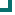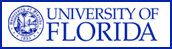Streptococcus pneumoniae (SOUTHEAST Data) 2004 2005 2006 2007 2008 2009 2010 penicillin 51.6%n=657 48.8%n=763 50%n=488 53%n=549 46.8%n=325 54.8%n=124 53.2%n=94 amoxicillin/clavulanate 97.7%n=87 95.5%n=88 96.8%n=154 96.1%n=179 93.1%n=29 81.3%n=107 86.2%n=94 ampicillin 88%n=25 100%n=87 vancomycin 100%n=587 100%n=680 100%n=477 100%n=505 100%n=533 100%n=112 100%n=94 cefaclor 60.9%n=23 66.7%n=63 cefuroxime 69.2%n=214 67.2%n=119 63.5%n=271 67.2%n=232 53.8%n=199 70.2%n=124 60.6%n=94 cefotaxime 89.4%n=236 80.2%n=227 81.6%n=87 84.7%n=131 87.8%n=49 80%n=125 74.5%n=94 ceftriaxone 90%n=482 88.7%n=653 88.1%n=427 84.6%n=403 83.6%n=305 80%n=125 76.6%n=94 clindamycin 98.3%n=59 99%n=97 98.1%n=54 78.9%n=90 88.6%n=88 67.3%n=107 74.5%n=94 erythromycin 65.7%n=356 63.1%n=452 62.2%n=188 65.1%n=209 54.7%n=362 40.2%n=107 40.4%n=94 azithromycin 55.9%n=68 57%n=93 55.2%n=29 39.8%n=108 40.4%n=94 levofloxacin 98.7%n=377 98.8%n=426 97.1%n=278 99%n=305 97.8%n=360 99.2%n=125 97.9%n=94 gatifloxacin 98.3%n=59 100%n=66 98.1%n=54 98.2%n=56 96.2%n=26 TMP/SMX 63.6%n=349 67.4%n=380 62.4%n=229 64.7%n=266 58.6%n=324 37.1%n=124 57.4%n=94 chloramphenicol 94.3%n=35 93.2%n=221 96%n=25 90.6%n=64 92.3%n=78 Totals 79.5%n=3498 79.3%n=4172 78.1%n=2848 79%n=3232 75.2%n=2629 69.8%n=1366 69.2%n=1034

This data is accurate as of: 11/22/2016 11:53:58 AM

Drugs with less than 10 isolates are removed from the chart and from the graph. The following drugs had some data removed: amoxicillin/clavulanate, cefazolin, clindamycin, gatifloxacin, chloramphenicol, ampicillin, cefaclor, cefotaxime, azithromycin, penicillin, vancomycin, cefuroxime, ceftriaxone, erythromycin, levofloxacin, TMP/SMX.ARMProgram.com is a project run by the University of Florida. Copyright 2011. All Rights Reserved. Site by JMarc Technologies, LLC